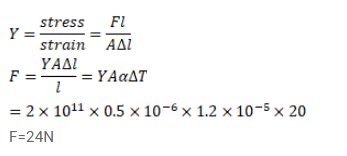# A steel wire of cross-sectional area`
Question:

A steel wire of cross-sectional area $0.5 \mathrm{~mm}^{2}$ is held between two fixed supports. If the wire is just taut at $20^{\circ} \mathrm{C}$, determine the tension when the temperature falls to $0^{\circ} \mathrm{C}$. Coefficient of linear expansion of steel is $1.2^{\times 10^{-5} /{ }^{\circ} \mathrm{C}}$ and its Young'smodulus is $2.0^{\times 10^{11}} \mathrm{~N} / \mathrm{m}^{2}$

Solution: